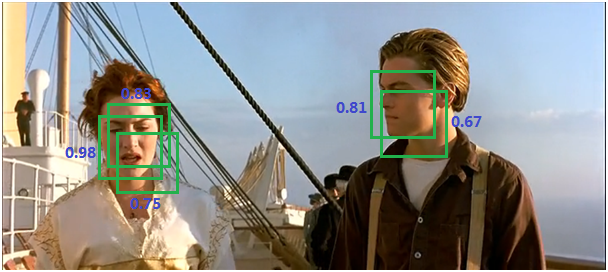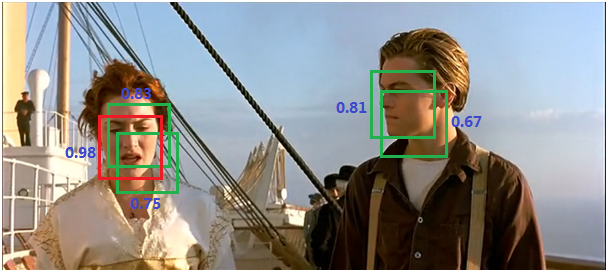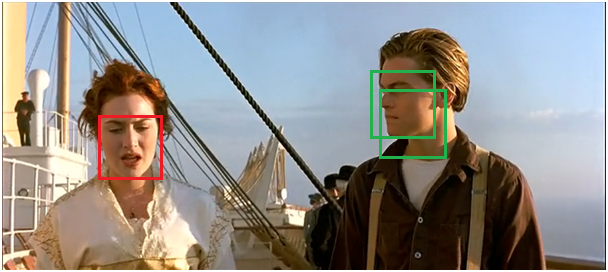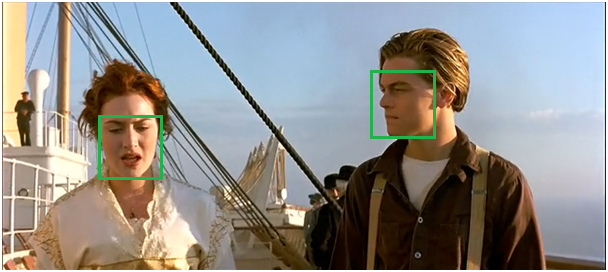# NMS——非极大值抑制

NMS（non maximum suppression），中文名非极大值抑制，在很多计算机视觉任务中都有广泛应用，如：边缘检测、目标检测等。

（1） 绝大部分人脸检测器的核心是分类器，即给定一个尺寸固定图片，分类器判断是或者不是人脸；

（2）将分类器进化为检测器的关键是：在原始图像上从多个尺度产生窗口，并resize到固定尺寸，然后送给分类器做判断。最常用的方法是滑动窗口。（1）将所有框的得分排序，选中最高分及其对应的框：（2）遍历其余的框，如果和当前最高分框的重叠面积(IOU)大于一定阈值，我们就将框删除。（3）从未处理的框中继续选一个得分最高的，重复上述过程。%% NMS:non maximum suppression
function pick = nms(boxes,threshold,type)
% boxes: m x 5,表示有m个框，5列分别是[x1 y1 x2 y2 score]
% threshold: IOU阈值
% type：IOU阈值的定义类型

% 输入为空，则直接返回
if isempty(boxes)
pick = [];
return;
end

% 依次取出左上角和右下角坐标以及分类器得分(置信度)
x1 = boxes(:,1);
y1 = boxes(:,2);
x2 = boxes(:,3);
y2 = boxes(:,4);
s = boxes(:,5);

% 计算每一个框的面积
area = (x2-x1+1) .* (y2-y1+1);

%将得分升序排列
[vals, I] = sort(s);

%初始化
pick = s*0;
counter = 1;

% 循环直至所有框处理完成
while ~isempty(I)
last = length(I); %当前剩余框的数量
i = I(last);%选中最后一个，即得分最高的框
pick(counter) = i;
counter = counter + 1;

%计算相交面积
xx1 = max(x1(i), x1(I(1:last-1)));
yy1 = max(y1(i), y1(I(1:last-1)));
xx2 = min(x2(i), x2(I(1:last-1)));
yy2 = min(y2(i), y2(I(1:last-1)));
w = max(0.0, xx2-xx1+1);
h = max(0.0, yy2-yy1+1);
inter = w.*h;

%不同定义下的IOU
if strcmp(type,'Min')
%重叠面积与最小框面积的比值
o = inter ./ min(area(i),area(I(1:last-1)));
else
%交集/并集
o = inter ./ (area(i) + area(I(1:last-1)) - inter);
end

%保留所有重叠面积小于阈值的框，留作下次处理
I = I(find(o<=threshold));
end
pick = pick(1:(counter-1));
end